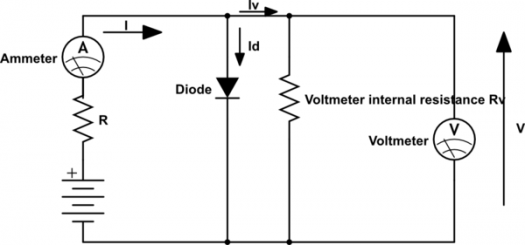# Quiz: Diode Resistance And Electric Circuit Test! Trivia Questions

20 Questions | Total Attempts: 50SettingsA diode according to our physics class, is a semiconductor device that allows electric correct to pass in one direction while blocking its flow in the opposite direction. The ideal diode does not restrict the movement of the current forward, and it can completely bar the reverse voltage polarity. Test your understanding of diode resistance in the electric circuit by taking the test below.

Related Topics
• 1.
Which of the following statement is false for a Zener diode voltage rated as “VZ”?
• A.

Reverse breakdown occurs at VZ

• B.

Forward breakdown occurs at VZ

• C.

Zener diode is a heavily doped diode

• D.

Usually operates in reverse bias mode

• 2.
After cut-in voltage AC resistance of the diode
• A.

Slightly decreases

• B.

Decreases exponentially

• C.

Slightly increases

• D.

Increases exponentially

• 3.
Determine DC resistance of a diode described by the characteristic graph at ID = 10mA.
• A.

9 Ω

• B.

11.11 Ω

• C.

10.10 Ω

• D.

13.13 Ω

• 4.
Which type of circuit is given in the diagram?
• A.

Clipper

• B.

Clamper

• C.

Rectifier

• D.

None of the mentioned

• 5.
Negative resistance are incorporated in oscillator for____________
• A.

Sustained oscillation

• B.

Damped oscillation

• C.

Biasing the oscillator

• D.

Increasing amplitude of oscillation

• 6.
Which of the following correctly determines the relation between α and β?
• A.

β=α/(1-α)

• B.

α=β/(1/α)

• C.

β=α/(1-β)

• D.

None of the mentioned

• 7.
For common emitter configuration, which of the following is the correct relation?
• A.

IC < IE

• B.

IC=βIB

• C.

IC =αIE

• D.

All of the mentioned

• 8.
Considering the resistance of the emitter, collector and base to be Re, Rc and Rb respectively, which of the following is the correct statements?
• A.

Re > Rb

• B.

Rc > Rb > Re

• C.

Rb > Rc > Re

• D.

None of the mentioned

• 9.
• A.

It has no enable input.

• B.

It has an invalid state.

• C.

It has no clock input.

• D.

It has only a single output.

• 10.
How many flip-flops are required to make a MOD-32 binary counter?
• A.

3

• B.

45

• C.

5

• D.

6

• 11.
Convert the binary number 1001.00102 to decimal.
• A.

90.125

• B.

9.125

• C.

125

• D.

12.5

• 12.
The carry propagation can be expressed as ______________
• A.

Cp = AB

• B.

Cp = A + B

• C.

Cp = A - B

• D.

Cp = A | B

• 13.
The bit sequence 0010 is serially entered (right-most bit first) into a 4-bit parallel out shift register that is initially clear. What are the Q outputs after two clock pulses?
• A.

0000

• B.

0010

• C.

1000

• D.

1111

• 14.
Computers operate on data internally in a format.
• A.

Tri-state

• B.

Universal

• C.

Parallel

• D.

Serial

• 15.
Another name for a unity gain amplifier is ____________________
• A.

Difference amplifier

• B.

Comparator

• C.

Single ended

• D.

Voltage follower

• 16.
When a capacitor is used in place of a resistor in an op-amp network, its placement determines ________________________
• A.

Open- or closed-loop gain

• B.

Integration or differentiation

• C.

Saturation or cut-off

• D.

• 17.
In a low pass butterworth filter, the condition at which f=fH is called __________
• A.

Cut-off frequency

• B.

Break frequency

• C.

Corner frequency

• D.

All of the mentioned

• 18.
Zero crossing detectors is also called as _______________________
• A.

Square to sine wave generator

• B.

Sine to square wave generator

• C.

Sine to triangular wave generator

• D.

All of the mentioned

• 19.
Which circuit converts irregularly shaped waveform to regular shaped waveforms?
• A.

Schmitt trigger

• B.

Voltage limiter

• C.

Comparator

• D.

None of the mentioned

• 20.
Number of pins in IC μA741 __________________
• A.

8

• B.

6

• C.

4

• D.

None